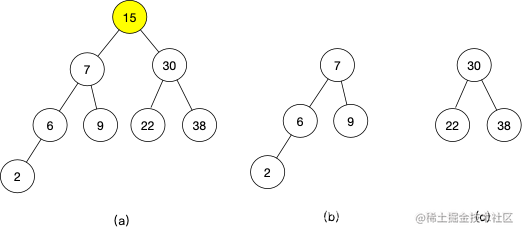# 二分查找思想的迁移应用

## 求根号x

class Solution {
public int search(int[] nums, int target) {
int left = 0;
int right = nums.length - 1;
while (left <= right) {
int mid = (right - left) / 2 + left;
if (nums[mid] == target) {
return mid;
} else if (nums[mid] < target) {
left = mid + 1;
} else {
right = mid - 1;
}
}
return -1;
}
}

class Solution {
public int mySqrt(int x) {
if (x == 0)
return 0;
int left = 1, right = x;
while(true) {
int mid = (right - left) / 2 + left;
if (mid > x / mid) {
right = mid - 1;
} else {
if ((mid + 1) > x / (mid + 1)) {
return mid;
}
left = mid + 1;
}
}
}
}

## 二叉搜索树中第K小的元素class Solution {
public int kthSmallest(TreeNode root, int k) {
int n = count(root.left);
if (k <= n) {
return kthSmallest(root.left, k);
} else if (k > n + 1) {
return kthSmallest(root.right, k - n - 1);
}
return root.val;
}

private int count(TreeNode root) {
if (root == null) {
return 0;
}
return 1 + count(root.left) + count(root.right);
}
}

## 寻找重复数

f(1) = 1;
f(2) = 2;
f(3) = 3;
f(4) = 5;
f(5) = 6;
f(6) = 7;

$\begin{cases} f(x)\le x，&\text{对于 }x x，&\text{对于 }x\ge d \end{cases}$

• 第一步：left = 1， right = 6，mid = 3，数组中小于等于mid的个数为3，符合公式(1),说明重复元素在[mid+1, rihgt]这个区间；
• 第二步：left = 4，right = 6，mid = 5，数组中小于等于mid的个数为6，符合公式（2），说明重复元素在[left, mid]这个区间；
• 第三步：left = 4，right = 5，mid = 4，数组中小于等于mid的个数为5，符合公式（2），说明重复元素在[left, mid]这个区间；
• 第四步：由于left = right，结束，答案为4。

class Solution {
public int findDuplicate(int[] nums) {
int left = 1, right = nums.length - 1;
while (left < right) {
int mid = (right - left) / 2 + left;
int cnt = 0;
for (int v : nums) {
if (v <= mid) {
cnt++;
}
}
if (cnt <= mid) {
left = mid + 1;
} else {
right = mid;
}
}
return left;
}
}